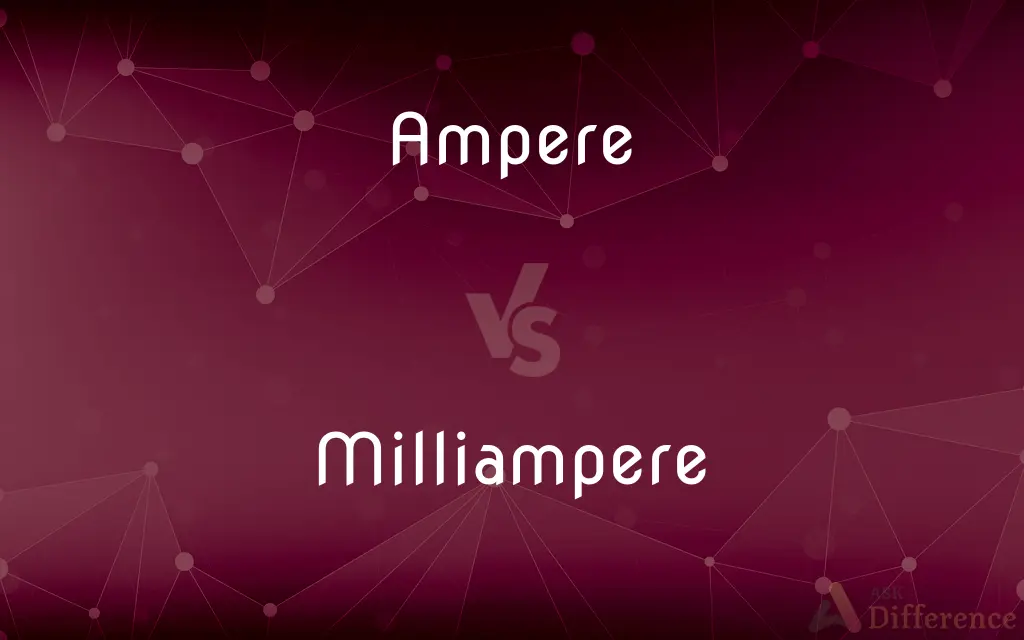# Ampere vs. Milliampere — What's the Difference?## Definitions

#### Ampere➦

The ampere (, US: ; symbol: A), often shortened to amp, is the base unit of electric current in the International System of Units (SI). It is named after André-Marie Ampère (1775–1836), French mathematician and physicist, considered the father of electromagnetism.

#### Milliampere➦

A unit of current equal to one thousandth (10-3) of an ampere.

#### Ampere➦

The basic unit of electric current, equal to one coulomb per second and equivalent to the current, flowing in two straight parallel wires of negligible cross section separated by a distance of one meter, that produces a force between the wires of 2.0 × 10-7 newtons per meter of length. The value of an ampere in the International System differs very slightly from that in the meter-kilogram-second-ampere system of units. See Table at measurement.

#### Milliampere➦

One thousandth (10-3) of an ampere.

#### Ampere➦

A unit of electrical current, the standard base unit in the International System of Units; colloquially amp. Abbreviation: amp, Symbol: A
Definition: The ampere, symbol A, is the SI unit of electric current. It is defined by taking the fixed numerical value of the elementary charge e to be 1.602
X 10−19 when expressed in the unit C, which is equal to A
S, where the second is defined in terms of ΔνCs. ([https://www.bipm.org/en/si-base-units/ampere The International Bureau of Weights and Measures])

#### Milliampere➦

The thousandth part of one ampère.

#### Ampere➦

The unit of electric current; - defined by the International Electrical Congress in 1893 and by U. S. Statute as, one tenth of the unit of current of the C. G. S. system of electro-magnetic units, or the practical equivalent of the unvarying current which, when passed through a standard solution of nitrate of silver in water, deposits silver at the rate of 0.001118 grams per second. Called also the international ampère.

#### Milliampere➦

One thousandth of an ampere

#### Ampere➦

A former unit of electric current (slightly smaller than the SI ampere)

#### Ampere➦

The basic unit of electric current adopted under the Systeme International d'Unites;
A typical household circuit carries 15 to 50 amps